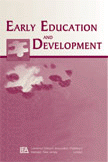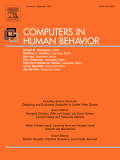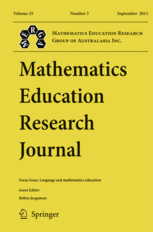# Math Shelf eliminates early math achievement gaps

0
Number of months Math Shelf students outperformed comparison students
0
Percentile point math gain for Math Shelf students versus the district average
0
Percentage of Math Shelf students that master state and common core standards
0
Number of games the average Math Shelf classroom plays per year

# Read Math Shelf peer-reviewed studiesIn a randomized controlled trial, children that played Math Shelf learned six months more math than students that played the five most downloaded and best reviewed math apps in 2014. Read the study in Early Education and Development.English Language Learners that played Math Shelf for 18 weeks learned one year more math than students that received classroom mathematics instruction. Read the study in Computers in Human Behavior.In a large-scale randomized trial, 500 children that played Math Shelf learned nine months more math than students that were taught a research-based hands on mathematics curriculum. Read the article in the Mathematics Education Research Journal.

# The sooner we make early math inequities a priority, the sooner we can eliminate disparities in educational outcomes

• Kindergarten mathematics knowledge is the best predictor of high school academic success
(Watts et al., 2014).
• Preschool mathematics knowledge predicts adults' earnings potential
(Geary et al., 2013).
• Preschool mathematics knowledge is a better predictor of elementary school academic success than literacy readiness skills
(Duncan et al., 2007).